Students can download 12th Business Maths Chapter 2 Integral Calculus I Ex 2.3 Questions and Answers, Samacheer Kalvi 12th Business Maths Book Solutions Guide Pdf helps you to revise the complete Tamilnadu State Board New Syllabus and score more marks in your examinations.

## Tamilnadu Samacheer Kalvi 12th Business Maths Solutions Chapter 2 Integral Calculus I Ex 2.3

Integrate the following with respect to x.

Question 1.
$$e^{x \log a}+e^{a \log a}-e^{n \log x}$$
Solution: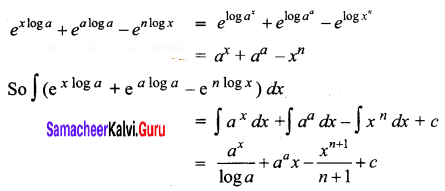Question 2.
$$\frac{a^{x}-e^{x \log b}}{e^{x \log a} b^{x}}$$
Solution: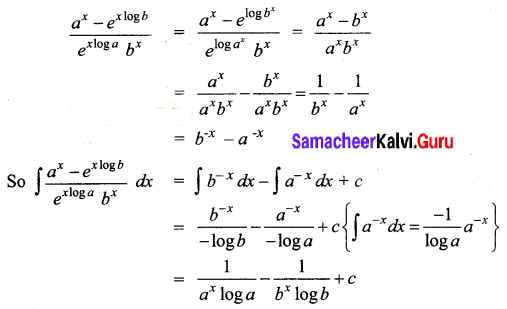Question 3.
$$\left(e^{x}+1\right)^{2} e^{x}$$
Solution: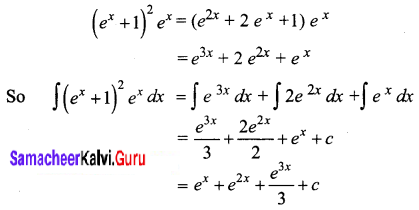Question 4.
$$\frac{e^{3 x}-e^{-3 x}}{e^{x}}$$
Solution: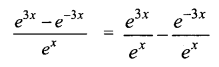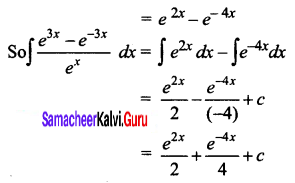Question 5.
$$\frac{e^{3 x}+e^{5 x}}{e^{x}+e^{-x}}$$
Solution: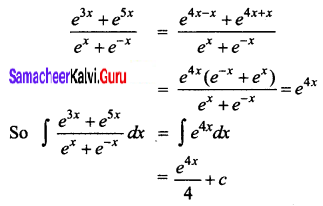Question 6.
$$\left[1-\frac{1}{x^{2}}\right] e^{\left(x+\frac{1}{x}\right)}$$
Solution: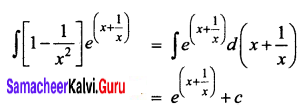Question 7.
$$\frac{1}{x(\log x)^{2}}$$
Solution: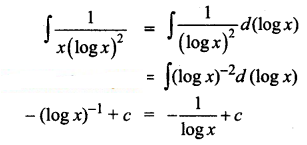Question 8.
If f'(x) = ex and f(0) = 2, then find f(x).
Solution:
f'(x) = ex
Integrating both sides of the equation,
∫ f'(x) dx = ∫ ex dx
⇒ f(x) = ex + c
given f(0) = 2
2 = e0 + c
⇒ c = 1
Thus f(x) = ex + 1